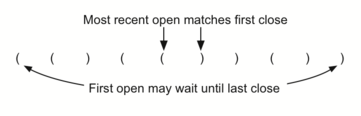3.6. Simple Balanced Parentheses¶

We now turn our attention to using stacks to solve real computer science problems. You have no doubt written arithmetic expressions such as

$$(5+6)*(7+8)/(4+3)$$

where parentheses are used to order the performance of operations. You may also have some experience programming in a language such as Lisp or Scheme with constructs like:

(defun square(n)
(* n n))

This defines a function called square that will return the square of its argument n. Scheme and Lisp are both notorious for using lots and lots of parentheses.

In both of these examples, parentheses must appear in a balanced fashion. Balanced parentheses means that each opening symbol has a corresponding closing symbol and the pairs of parentheses are properly nested. Consider the following correctly balanced strings of parentheses:

(()()()())

(((())))

(()((())()))

Compare those with the following, which are not balanced:

((((((())

()))

(()()(()

The ability to differentiate between parentheses that are correctly balanced and those that are unbalanced is an important part of recognizing many programming language structures.

The challenge then is to write an algorithm that will read a string of parentheses from left to right and decide whether the symbols are balanced. To solve this problem we need to make an important observation. As you process symbols from left to right, the most recent opening parenthesis must match the next closing symbol (see Figure 4). Also, the first opening symbol processed may have to wait until the very last symbol for its match. Closing symbols match opening symbols in the reverse order of their appearance; they match from the inside out. This is a clue that stacks can be used to solve the problem.Figure 4: Matching Parentheses

Once you agree that a stack is the appropriate data structure for keeping the parentheses, the statement of the algorithm is straightforward. Starting with an empty stack, process the parenthesis strings from left to right. If a symbol is an opening parenthesis, push it on the stack as a signal that a corresponding closing symbol needs to appear later. If, on the other hand, a symbol is a closing parenthesis, pop the stack. As long as it is possible to pop the stack to match every closing symbol, the parentheses remain balanced. If at any time there is no opening symbol on the stack to match a closing symbol, the string is not balanced properly. At the end of the string, when all symbols have been processed, the stack should be empty. The C++ and Python code to implement this algorithm is shown in ActiveCode 1.

This function, parChecker, assumes that a Stack class is available and returns a Boolean result as to whether the string of parentheses is balanced. Note that the Boolean variable balanced is initialized to true` as there is no reason to assume otherwise at the start. If the current symbol is (, then it is pushed on the stack (lines 15-16). Note also in line 22 that pop simply removes a symbol from the stack. The returned value is not used since we know it must be an opening symbol seen earlier. At the end (lines 28-31), as long as the expression is balanced and the stack has been completely cleaned off, the string represents a correctly balanced sequence of parentheses.